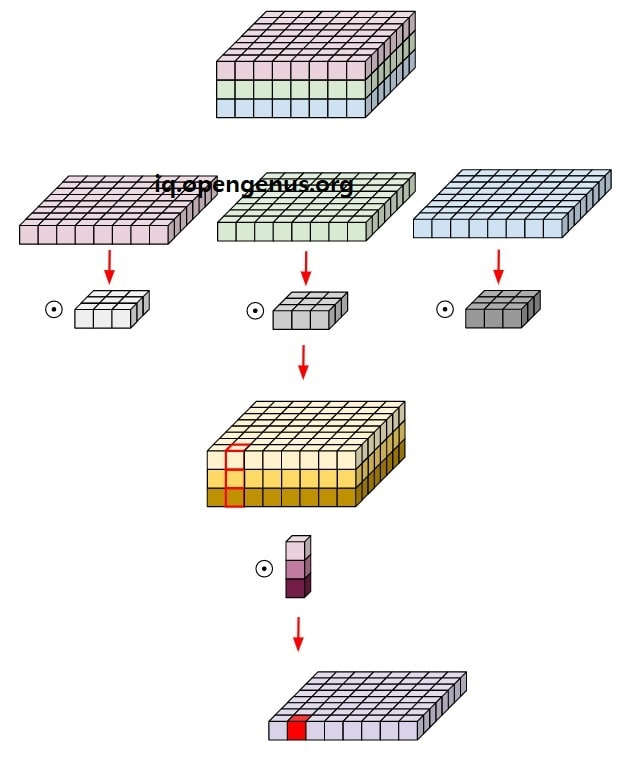# Depthwise Convolution

Get FREE domain for 1st year and build your brand new siteDepthwise Convolution is a special case of Group Convolution where number of input channel is same as number of output channels. It reduces the number of floating point operations nearly by a factor of number of channels compared to the standard Convolution approach.

We have covered the following points regarding Depthwise Convolution in depth:

• Floating point operations in Depthwise Convolution
• Use of Depthwise Convolution
• Algorithm of Depthwise Convolution with pseudocode
• Depthwise Convolution in TensorFlow and OneDNN

# FLOP in Depthwise Convolution

The number of floating point operations is: 2 × bs x ic × oh × ow × (kh × kw + oc)

``````2 × bs x ic × oh × ow × (kh × kw + oc)
``````

where the parameters are:

• bs: Batch size
• ic: Input channel
• oh: Output height
• ow: Output width
• kh: kernel height
• kw: kernel width
• oc: Output channel

The number of floating point operations in standard Convolution takes:

``````2 × bs x ic × oh × ow × kh × kw x oc
``````

where the parameters are:

• bs: Batch size
• ic: Input channel
• oh: Output height
• ow: Output width
• kh: kernel height
• kw: kernel width
• oc: Output channel

Notice that in the Depthwise Convolution, oc (Output channel) is added (+) while in the Standard Convolution, it is multiplied.

# Use of Depthwise Convolution

Depthwise Convolution is used in MobileNetV2 model.

In the research paper which introduced MobileNet, Depthwise Convolution is described as a separable Convolution which separates the input data across the channel dimension.

Depthwise Convolution was originally introduced in AlexNet where it was used to save memory.

# Steps in Depthwise Convolution

The general steps in Depthwise Convolution are:

• Split the input across channel dimension. We have ic inputs.
• Split the weights across channel dimension. We have ic weights.
• Do dot product of i-th weight with i-th input. We have ic intermediate inputs.
• Merge the ic intermediate data.

You shall visualize the process as follows:# Pseudocode of Depthwise Convolution

The Pseudocode of Depthwise Convolution is as follows:

``````depthwise_conv2d()
# input: (height, width, channel)
# weight: (filter_dimension, filter_dimension, channel)
# Output: (o_height, o_width, channel)

assert weight.shape == weight.shape and weight.shape % 2 == 1

height, width, channel = input.shape

output = (o_height, o_width, channel) [Empty]

for ch from 0 to (channel):
for i from 0 to (height):
for j from 0 to (width):
for filter_i from 0 to (w.shape):
for filter_j from 0 to (w.shape):
weight_element = w[filter_i, filter_j, ch]
output[i][j][c] += padded_input[i + filter_i][j + filter_j][c] * weight_element
``````

# Depthwise Convolution in TensorFlow

In TensorFlow, Depthwise Convolution is a special case of Separable Convolution tf.nn.separable_conv2d. The condition is number of input channel is same as number of output channel.

``````tf.nn.separable_conv2d
``````

The function parameters of this call is as follows:

``````tf.nn.separable_conv2d(
input, depthwise_filter, pointwise_filter, strides, padding, data_format=None,
dilations=None, name=None
)
``````

# Depthwise Convolution in OneDNN

Following are the data involved in depthwise convolution:

• Input [batch_size, channels, input_height, input_width]
• Kernel size [kernel_height, kernel_width]
• Output [batch_size, channel, output_height, output_width]

To use Depthwise Convolution in OneDNN, we need to to ensure input channel is same as output channel and create the weight descriptor as a 5D vector.

``````source_descriptor = {{batch_size, channels, input_height, input_width}, f32, any};
weight_descriptor = {{
/* groups = */ channels,
/* oc =  */ 1,  /* ic = */ 1,
/* kh = */ kernel_height,
/* kw = */ kernel_width},
f32, any};
destination_descriptor = {{batch_size, channels, output_height, output_width}, f32, any};
// create convolution as usual
``````

# Conclusion

Depthwise Convolution is an efficient convolution algorithm where applicable and reduces the number of floating point operations significantly. This brings in significant performance improvement in Deep Learning softwares like TensorFlow and OneDNN.

``````Benjamin QoChuk, OpenGenus Foundation.